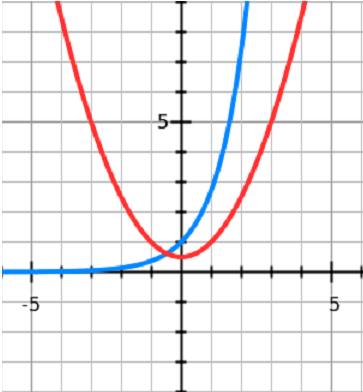# Find the no of solutions of the equation2^x = x^2 + 1Caution : answer is not 2 ;)

9 years ago

2^x = x^2 + 1

Take log both sides with base ''e''

xlog2 = log(x^2 + 1)

x = log(x^2 + 1)/log2

x = log[(x^2 + 1)/(2)]

(x^2 + 1)/(2) = e^x

x^2/2 + 1/2   = e^xFrom this graph we conclude that both graph cuts each other at only one point.

Hence there is onle and only one solution.

Plz Approve!

9 years ago

No no no see
u will surely get two values 1 and 0
Now if u take f(x)=x^2 + 1 - 2^x
find f''(x) now
apply rolles theorem
u will get that f(x)=0 has 3 roots
another root will be b/w 4 and 5